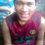# Weird!!!

Find the value of $\sum\limits_{x=-2013}^{2013} x \cdot |x| \cdot \lfloor x \rfloor \cdot \text {sgn} (x)$.

Here, $\lfloor x \rfloor$ denotes the greatest integer function or floor function, and $\text {sgn} (x)$ denotes the sign function: 1 if $x$ is positive, 0 if $x$ is zero, and -1 if $x$ is negative.

I have invented this question, and got the answer $\boxed{0}$ (correct me if I'm wrong), but I don't know how to solve this without using graphs. Please help. Thanks.Note by Jaydee Lucero
6 years, 10 months ago

This discussion board is a place to discuss our Daily Challenges and the math and science related to those challenges. Explanations are more than just a solution — they should explain the steps and thinking strategies that you used to obtain the solution. Comments should further the discussion of math and science.

When posting on Brilliant:

• Use the emojis to react to an explanation, whether you're congratulating a job well done , or just really confused .
• Ask specific questions about the challenge or the steps in somebody's explanation. Well-posed questions can add a lot to the discussion, but posting "I don't understand!" doesn't help anyone.
• Try to contribute something new to the discussion, whether it is an extension, generalization or other idea related to the challenge.

MarkdownAppears as
*italics* or _italics_ italics
**bold** or __bold__ bold
- bulleted- list
• bulleted
• list
1. numbered2. list
1. numbered
2. list
Note: you must add a full line of space before and after lists for them to show up correctly
paragraph 1paragraph 2

paragraph 1

paragraph 2

[example link](https://brilliant.org)example link
> This is a quote
This is a quote
    # I indented these lines
# 4 spaces, and now they show
# up as a code block.

print "hello world"
# I indented these lines
# 4 spaces, and now they show
# up as a code block.

print "hello world"
MathAppears as
Remember to wrap math in $$ ... $$ or $ ... $ to ensure proper formatting.
2 \times 3 $2 \times 3$
2^{34} $2^{34}$
a_{i-1} $a_{i-1}$
\frac{2}{3} $\frac{2}{3}$
\sqrt{2} $\sqrt{2}$
\sum_{i=1}^3 $\sum_{i=1}^3$
\sin \theta $\sin \theta$
\boxed{123} $\boxed{123}$

Sort by:

Note that $\lfloor x \rfloor = x$ as $x$ is an integer, and $|x| \cdot \text{sgn}(x) = x$ for all $x$. So you're basically asking $\displaystyle\sum_{x=-2013}^{2013} x^3$, which is now easy to solve.

- 6 years, 10 months ago

Precise! So 0 is correct answer.

- 6 years, 10 months ago

To evaluate the sum, note that terms of opposite signs cancel, and the only term left is $0^3 = 0.$

- 6 years, 10 months ago

now I see... thanks... :D

- 6 years, 10 months ago

by graph: The above function takes values of x on the X-axis and y=f(x) on the Y axis. this question is basically to plot the graph of f(x)=x^3, here we are only taking the integers values at x-axis and getting correspond y values as integers.So instead of smooth curve we will will be having the points which lies on the curve of f(x)=x^3.

- 6 years, 10 months ago